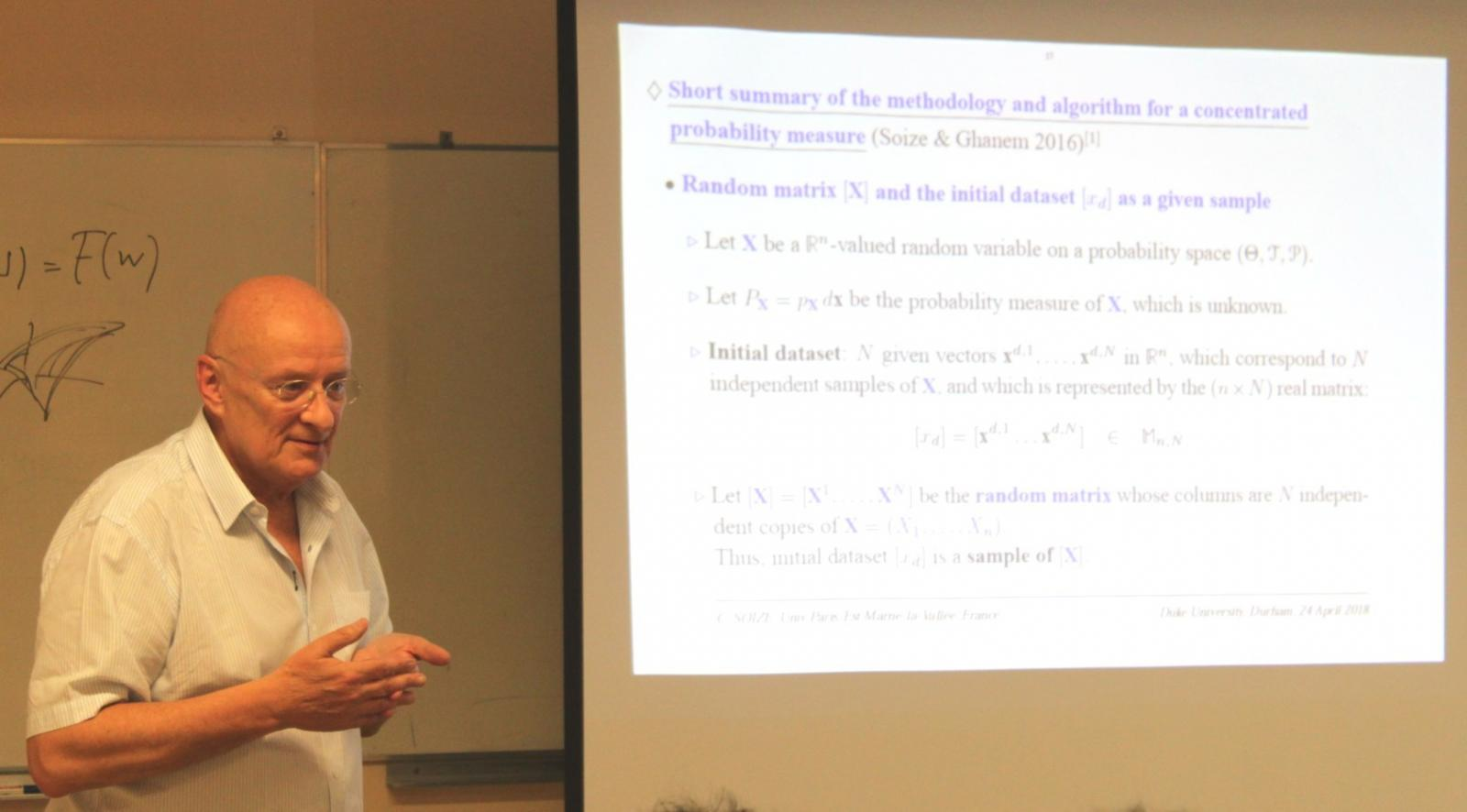# Probabilistic learning in computational mechanics

Au LMSSC, Paris, le 31 mai 2018 à 14h

Christian Soize
Professeur des Universités, Laboratoire de Modélisation et Simulation Multi Échelle (MSME),
Université Paris-Est Marne-la-Vallée (UPEM), FranceThe talk will be devoted to the presentation of a novel approach concerning data driven and probabilitistic learning on manifolds with applications in computational mechanics. This tool of the computational statistics can be viewed as a useful method in scientific machine learning based on the probability theory.

We first explaining the concept/method of this probabilistic learning on manifolds by discussing a challenging problem of nonconvex optimization under uncertainties (OUU). We will then present the mathematical formulation and the main steps of the method based on the construction of a diffusion-maps basis and the projection on it of a nonlinear Itô stochastic differential equation.

After having presented two simple illustrations, fours applications will be presented:

• Optimization under uncertainties using a limited number of function evaluations.
• Enhancing model predictability for a scramjet using probabilistic learning on manifolds.
• Design optimization under uncertainties of a mesoscale implant in biological tissues using probabilistic learning.
• Probabilistic learning on manifolds for nonparametric probabilistic approach of model-form uncertainties in nonlinear computational mechanics.

Laboratoire de Mécanique des Structures et des Systèmes Couplés - LMSSC